• ## 彻底理解0-1背包问题

万次阅读 多人点赞 2018-10-07 08:33:04
0-1背包问题 给定n个重量为w1，w2,w3,…,wn，价值为v1,v2,v3,…,vn的物品和容量为C的背包，求这个物品中一个最有价值的子集，使得在满足背包的容量的前提下，包内的总价值最大 0-1背包问题指的是每个物品只能使用一...
0-1背包问题
给定n个重量为$w_1$，$w_2$,$w_3$,…,$w_n$，价值为$v_1$,$v_2$,$v_3$,…,$v_n$的物品和容量为$C$的背包，求这个物品中一个最有价值的子集，使得在满足背包的容量的前提下，包内的总价值最大
0-1背包问题指的是每个物品只能使用一次
递归方法
首先我们用递归的方式来尝试解决这个问题
我们用$F(n,C)$表示将前$n$个物品放进容量为$C$的背包里，得到的最大的价值。
我们用自顶向下的角度来看，假如我们已经进行到了最后一步（即求解将$n$个物品放到背包里获得的最大价值），此时我们便有两种选择

不放第$n$个物品，此时总价值为$F(n-1,C)$
放置第$n$个物品，此时总价值为$v_n+F(n-1,C-w_n)$

两种选择中总价值最大的方案就是我们的最终方案，递推式（有时也称之为状态转移方程）如下
$F(i,C)=max(F(i-1,C),v(i)+F(i-1,C-w(i)))$
编程实现如下：
public class KnapSack01 {
/**
* 解决背包问题的递归函数
*
* @param w        物品的重量数组
* @param v        物品的价值数组
* @param index    当前待选择的物品索引
* @param capacity 当前背包有效容量
* @return 最大价值
*/
private static int solveKS(int[] w, int[] v, int index, int capacity) {
//基准条件：如果索引无效或者容量不足，直接返回当前价值0
if (index < 0 || capacity <= 0)
return 0;

//不放第index个物品所得价值
int res = solveKS(w, v, index - 1, capacity);
//放第index个物品所得价值（前提是：第index个物品可以放得下）
if (w[index] <= capacity) {
res = Math.max(res, v[index] + solveKS(w, v, index - 1, capacity - w[index]));
}
return res;
}

public static int knapSack(int[] w, int[] v, int C) {
int size = w.length;
return solveKS(w, v, size - 1, C);
}

public static void main(String[] args){
int[] w = {2,1,3,2};
int[] v = {12,10,20,15};
System.out.println(knapSack(w,v,5));
}
}


记忆化搜索
我们用递归方法可以很简单的实现以上代码，但是有个严重的问题就是，直接采用自顶向下的递归算法会导致要不止一次的解决公共子问题，因此效率是相当低下的。
我们可以将已经求得的子问题的结果保存下来，这样对子问题只会求解一次，这便是记忆化搜索。
下面在上述代码的基础上加上记忆化搜索
public class KnapSack01 {
private static int[][] memo;

/**
* 解决背包问题的递归函数
*
* @param w        物品的重量数组
* @param v        物品的价值数组
* @param index    当前待选择的物品索引
* @param capacity 当前背包有效容量
* @return 最大价值
*/
private static int solveKS(int[] w, int[] v, int index, int capacity) {
//基准条件：如果索引无效或者容量不足，直接返回当前价值0
if (index < 0 || capacity <= 0)
return 0;

//如果此子问题已经求解过，则直接返回上次求解的结果
if (memo[index][capacity] != 0) {
return memo[index][capacity];
}

//不放第index个物品所得价值
int res = solveKS(w, v, index - 1, capacity);
//放第index个物品所得价值（前提是：第index个物品可以放得下）
if (w[index] <= capacity) {
res = Math.max(res, v[index] + solveKS(w, v, index - 1, capacity - w[index]));
}
//添加子问题的解，便于下次直接使用
memo[index][capacity] = res;
return res;
}

public static int knapSack(int[] w, int[] v, int C) {
int size = w.length;
memo = new int[size][C + 1];
return solveKS(w, v, size - 1, C);
}

public static void main(String[] args) {
int[] w = {2, 1, 3, 2};
int[] v = {12, 10, 20, 15};
System.out.println(knapSack(w, v, 5));
}
}


动态规划算法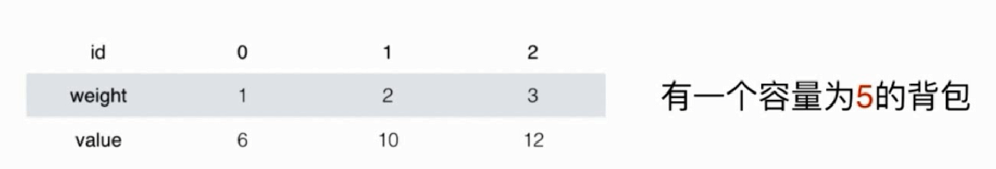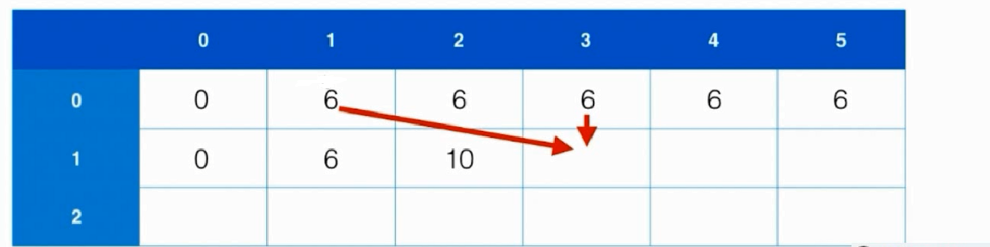public class KnapSack01 {
public static int knapSack(int[] w, int[] v, int C) {
int size = w.length;
if (size == 0) {
return 0;
}

int[][] dp = new int[size][C + 1];
//初始化第一行
//仅考虑容量为C的背包放第0个物品的情况
for (int i = 0; i <= C; i++) {
dp[i] = w <= i ? v : 0;
}
//填充其他行和列
for (int i = 1; i < size; i++) {
for (int j = 0; j <= C; j++) {
dp[i][j] = dp[i - 1][j];
if (w[i] <= j) {
dp[i][j] = Math.max(dp[i][j], v[i] + dp[i - 1][j - w[i]]);
}
}
}
return dp[size - 1][C];
}

public static void main(String[] args) {
int[] w = {2, 1, 3, 2};
int[] v = {12, 10, 20, 15};
System.out.println(knapSack(w, v, 5));
}
}


空间复杂度的极致优化
上面的动态规划算法使用了O(n*C)的空间复杂度（因为我们使用了二维数组来记录子问题的解），其实我们完全可以只使用一维数组来存放结果，但同时我们需要注意的是，为了防止计算结果被覆盖，我们必须从后向前分别进行计算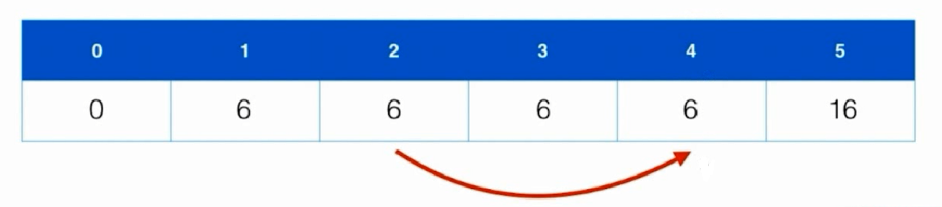我们仍然假设背包空间为5，根据$F(i,C)=max(F(i-1,C),v(i)+F(i-1,C-w(i)))$我们可以知道，当我们利用一维数组进行记忆化的时候，我们只需要使用到当前位置的值和该位置之前的值，举个例子
假设我们要计算$F(i,4)$,我们需要用到的值为$F(i-1,4)$和$F(i-1,4-w(i))$,因此为了防止结果被覆盖，我们需要从后向前依次计算结果
最终的动态规划代码如下
public class KnapSack01 {
public static int knapSack(int[] w, int[] v, int C) {
int size = w.length;
if (size == 0) {
return 0;
}

int[] dp = new int[C + 1];
//初始化第一行
//仅考虑容量为C的背包放第0个物品的情况
for (int i = 0; i <= C; i++) {
dp[i] = w <= i ? v : 0;
}

for (int i = 1; i < size; i++) {
for (int j = C; j >= w[i]; j--) {
dp[j] = Math.max(dp[j], v[i] + dp[j - w[i]]);
}
}
return dp[C];
}

public static void main(String[] args) {
int[] w = {2, 1, 3, 2};
int[] v = {12, 10, 20, 15};
System.out.println(knapSack(w, v, 5));
}
}


利用背包问题的思想解决问题
leetcode 416 Partition Equal Subset Sum

给定一个仅包含正整数的非空数组，确定该数组是否可以分成两部分，要求两部分的和相等

问题分析
该问题我们可以利用背包问题的思想进行求解。
假设给定元素个数为$n$的数组arr，数组元素的和为sum，对应于背包问题，等价于有$n$个物品，每个物品的重量和价值均为为arr[i]，背包的限重为sum/2，求解背包中的物品最大价值为多少？
class Solution {
private boolean knapSack(int[] nums,int sum){
int size = nums.length;

boolean[] dp = new boolean[sum + 1];
for (int i = 0;i <= sum;i ++){
dp[i] = i == nums;
}

for (int i = 1;i < size;i++){
for (int j = sum;j >= nums[i];j--){
dp[j] = dp[j] || dp[j-nums[i]];
}
}
return dp[sum];
}

public boolean canPartition(int[] nums) {
int sum = 0;
for (int item : nums){
sum += item;
}

//如果数组元素和不是2的倍数，直接返回false
if (sum % 2 != 0)
return false;

return knapSack(nums,sum/2);
}
}



展开全文动态规划
• 0-1背包问题解释及c++代码，0-1背包问题解释及c++代码，0-1背包问题解释及c++代码，0-1背包问题解释及c++代码
• 输入样例 输出样例 50 3 10 60 30 120 20 100 0 220 完整程序： #include<...// 0－1背包问题回溯算法实现 //（1） 0－1背包问题...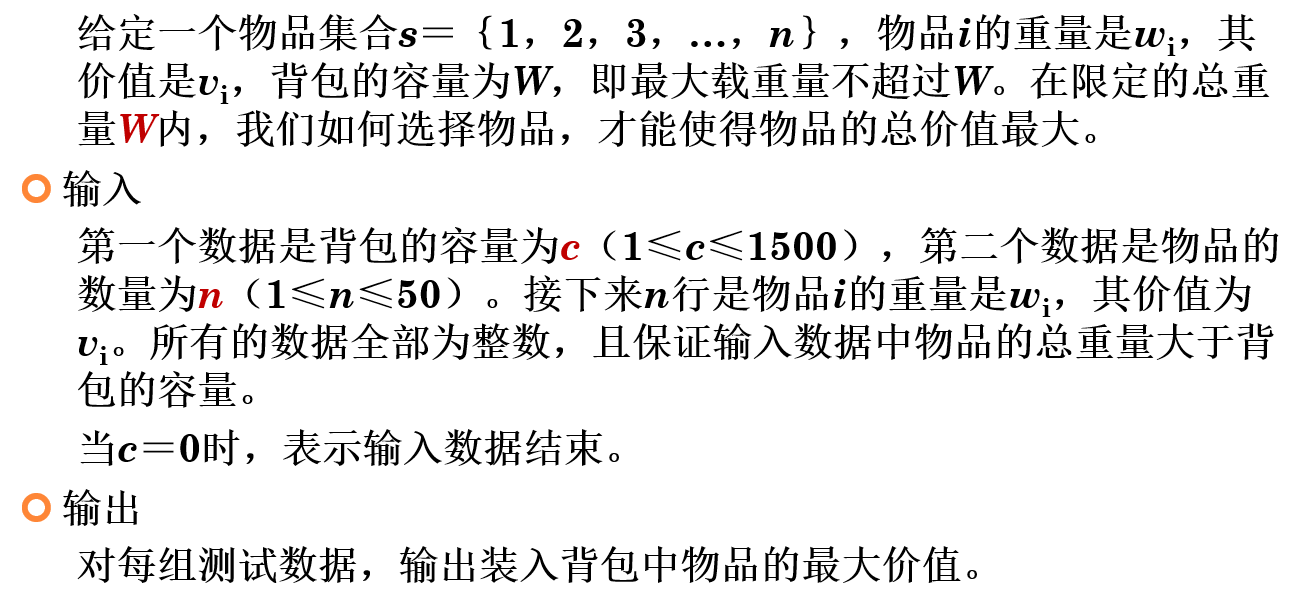输入样例

输出样例

50 3

10 60

30 120

20 100

0

220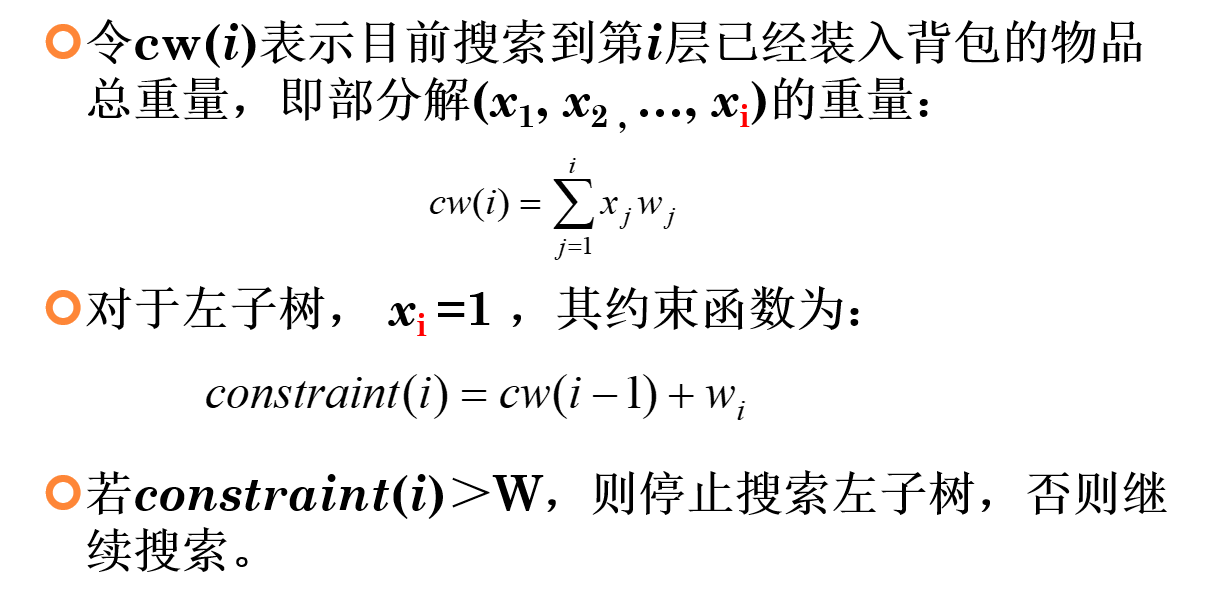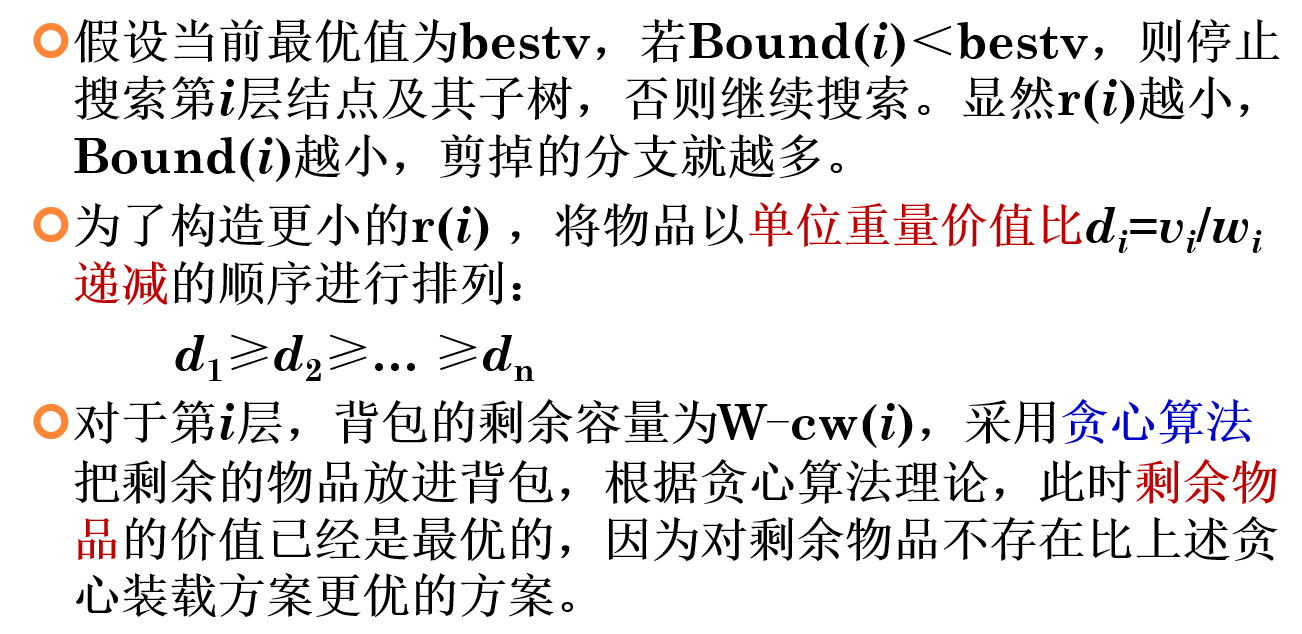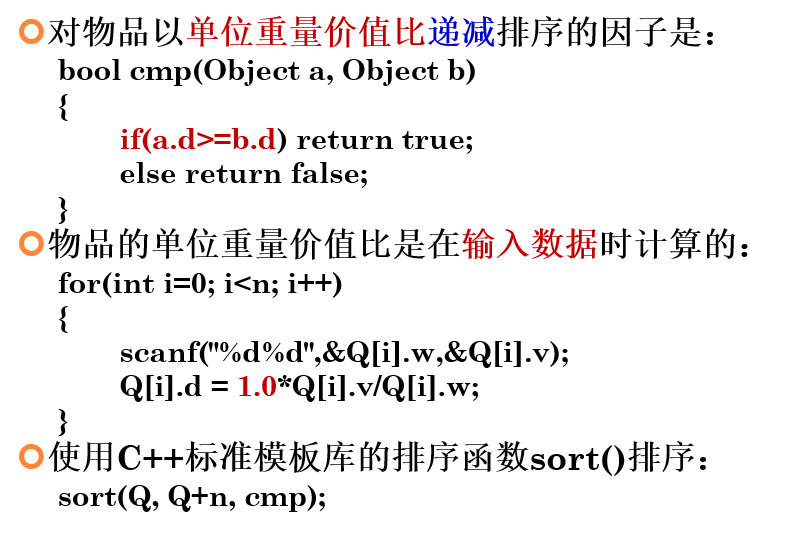完整程序：

#include<bits/stdc++.h>
using namespace std;
// 0－1背包问题回溯算法实现
//（1） 0－1背包问题回溯算法的数据结构
#define NUM 100
int c;				//背包的容量
int n;				//物品的数量
int cw;				//当前重量
int cv;				//当前价值
int bestv;			//当前最优价值
//描述每个物品的数据结构
struct Object{
int w;			//物品的重量
int v;			//物品的价值
double d;		//物品的单位重量价值比
}Q[NUM];			//物品的数组
//对物品以单位重量价值比递减排序的因子是：
bool cmp(Object a, Object b)
{
if(a.d>=b.d) return true;
else return false;
}
//（3）限界函数Bound()的实现
//形参i是回溯的深度
int Bound(int i)
{
int cleft=c-cw;//背包剩余的容量
int b=cv;//上界
//尽量装满背包
while(i<n&&Q[i].w<=cleft)
{
cleft-=Q[i].w;
b+=Q[i].v;
i++;
}
//剩余的部分空间也装满
if(i<n)b+=1.0*cleft*Q[i].v/Q[i].w;
return b;
}
//（2） 0－1背包问题回溯算法的实现
//形参i是回溯的深度，从0开始
void backtrack(int i)
{
//到达叶子结点时，更新最优值
if(i+1>n){bestv=cv;return;}
//进入左子树搜索
if(cw+Q[i].w<=c)
{
cw+=Q[i].w;
cv+=Q[i].v;
backtrack(i+1);
cw-=Q[i].w;
cv-=Q[i].v;
}
//进入右子树搜索
if(Bound(i+1)>bestv)backtrack(i+1);
}
int main(){
cout<<"请输入背包的容量和物品的数量"<<endl;
cin>>c;
while(c){
cin>>n;
cout<<"请依次输入物品的重量和价值"<<endl;
//物品的单位重量价值比是在输入数据时计算的：
for(int i=0; i<n; i++)
{
scanf("%d%d",&Q[i].w,&Q[i].v);
Q[i].d = 1.0*Q[i].v/Q[i].w;
}
//使用C++标准模板库的排序函数sort()排序：
sort(Q,Q+n,cmp);
backtrack(0);
cout<<"装入背包中物品的最大价值为："<<endl;
cout<<bestv<<endl;
cout<<"请输入背包的容量和物品的数量"<<endl;
cin>>c;
}
return 0;
}



展开全文• 首先看一波百度词条对于 “0 - 1背包问题” 的阐述： 01背包是在M件物品取出若干件放在空间为W的背包里，每件物品的体积为W1，W2至Wn，与之相对应的价值为P1,P2至Pn。01背包是背包问题中最简单的问题。01背包的约束...
什么是0 - 1背包
首先看一波百度词条对于 “0 - 1背包问题” 的阐述：
01背包是在M件物品取出若干件放在空间为W的背包里，每件物品的体积为W1，W2至Wn，与之相对应的价值为P1,P2至Pn。01背包是背包问题中最简单的问题。01背包的约束条件是给定几种物品，每种物品有且只有一个，并且有权值和体积两个属性。在01背包问题中，因为每种物品只有一个，对于每个物品只需要考虑选与不选两种情况。如果不选择将其放入背包中，则不需要处理。如果选择将其放入背包中，由于不清楚之前放入的物品占据了多大的空间，需要枚举将这个物品放入背包后可能占据背包空间的所有情况。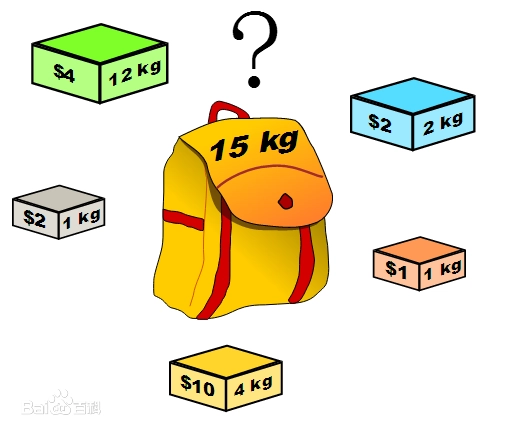是不是比较懵，那我们再对比地看一下 “背包问题” 帮助理解：
背包问题(Knapsack problem)是一种组合优化的NP完全问题。问题可以描述为：给定一组物品，每种物品都有自己的重量和价格，在限定的总重量内，我们如何选择，才能使得物品的总价格最高。问题的名称来源于如何选择最合适的物品放置于给定背包中。相似问题经常出现在商业、组合数学，计算复杂性理论、密码学和应用数学等领域中。也可以将背包问题描述为决定性问题，即在总重量不超过W的前提下，总价值是否能达到V？它是在1978年由Merkle和Hellman提出的。
简单来说背包问题就是能将物品分割成一部分装入背包（实现部分装入使得价值最大化），而 0 - 1背包呢，顾名思义，只有 0 （不装） 和 1（装入） 两种选择（这里我们讨论普通背包：一件物品只能被装入一次）；
解决实际问题
假设你现在有一个容量为8（指最多可装质量）的背包，摆在你面前的是一些有着不同质量且价值不等的物品，具体信息用数组表示为weight = [ 2, 3, 4, 5] , 对应价值 value = [ 6, 7, 6, 7]，现在的问题是如何装包使得价值最大化？
贪心思路
这里我们是不能使用贪心算法的，若我们使用一种贪心策略：始终选择当前物品中 rate = (value / weight) 最大的，也就是单位价值最大的物品。那么每种物品的单位价值为 unitVal = [ 3, 2.3, 1.5, 1.4]；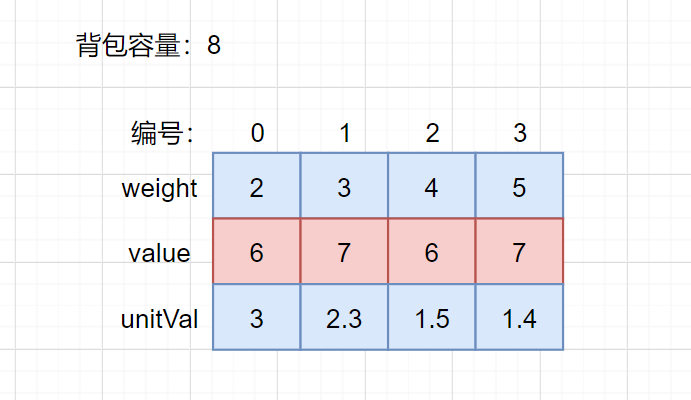据此我们首先选择 0号物品（编号从0开始），剩余容量为 8 - 2 = 6；紧接着选择 1号物品，背包剩余容量为 6 - 3 = 3;由于不可重复装包，剩下的两件物品我们无法再装入，得到最终结果 6 + 7 = 13，很显然这是不对的，因为我们可以选择1号与3号物品得到最大价值7 + 7 = 14；
动态规划求解
1. 确定dp数组以及下标的含义
这里涉及到背包容量c以及物品重量两个维度，我们使用二维数组来记录每一次选取得到的最大价值，二维数组的每一行表示一种物品，共计 weight.size() 行，数组的每一列表示背包的容量，共计 c （背包容量）+ 1列，dp[i][j]表示在编号从 i 到 最后一号物品所构成的物品集合中且背包容量为j时取得的最优解.即从 [i : 最后一号物品] 中任意取，放进容量为 j 的背包里，价值总和最大是多少。 如dp 表示 当背包容量为 5 时从 2 ~ 3 号物品选择出的最大价值；同理， dp 表示  当背包容量为 8 时从 1 ~ 3 号物品选择出的最大价值。
2. 确定递推公式
若当前背包容量不足以放下当前物品时（c < weight[i]）我们只能等于背包容量等于 c 且为装入当前物品时的最优解；而当背包容量可以放下当前物品时 （c >= weight[i]），我们有两种选择：装入当前物品 or 不装入；将当前物品装入后，背包还剩余的容量我们也能创造一定价值，这就要依赖dp数组中之前的解情况了，而选择不装入，就与上一种情况一样继承了之前的最优解，而我们需要两者的最大值。
因此dp公式为：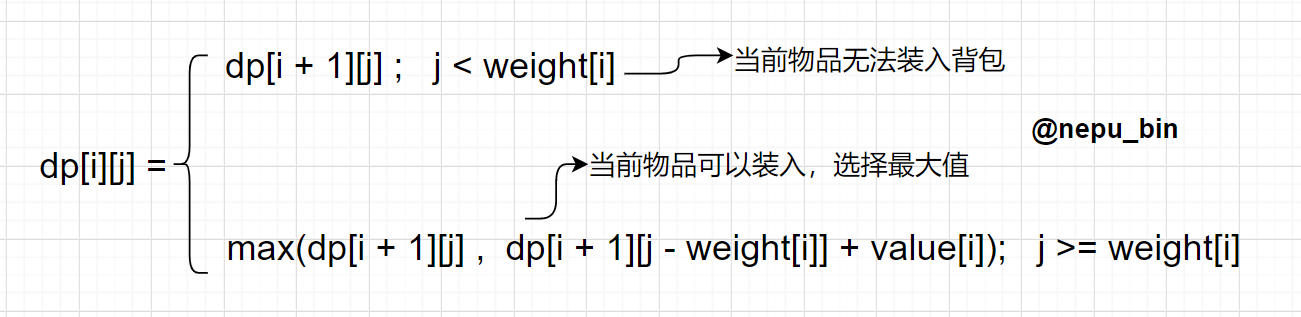3、dp数组如何初始化&&确定遍历顺序
根据第一点中叙述的dp数组的含义，当背包容量为0的时候不能装入任何物品，初始化 dp[i] = 0;
//注：row 为dp的行数，col 为dp的列数
for (int j = weight[row - 1]; j < col; ++j) {//当背包容量刚好放下最后一号物品时
//初始化dp数组
dp[rindex][j] = value[rindex];
}

遍历顺序：从dp数组最后一行从左往右自底向上(这里无特殊要求，行方向也可以从上往下遍历)
根据递推公式 dp[i][j] = max(dp[i + 1][j] ,  dp[i + 1][j - weight[i]] + value[i]) 以及遍历顺序，我们遍历第 i 个物品时可能需要用到 “i + 1” 个物品的解情况，因此我们遍历之前需要对dp数组最后一行进行初始化；
具体解释见代码；
dp数组详情（读者可自己根据递推公式推导）：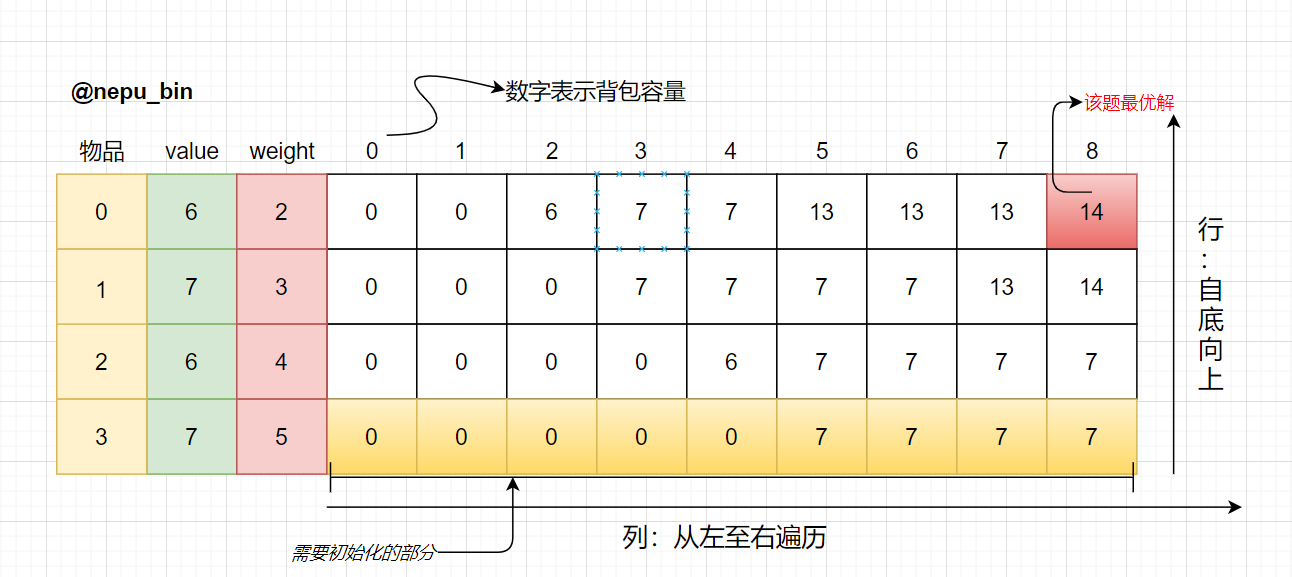#include <iostream>
#include <vector>
using namespace std;
//回溯背包装包过程，将状态记录到x数组中
void traceBack(vector<vector<int>>& dp, vector<int>& weight, vector<int>& x, int c) {
int len = x.size();
for (int i = 0; i < len - 1; i++) {
if (dp[i][c] == dp[i + 1][c])
x[i] = 0;
else {
x[i] = 1;
c -= weight[i];
}
}
//最后一行的装包情况，若大于0说明此物品被装入
x[len - 1] = (dp[len - 1][c] ? 1 : 0);
}

int ZeroOneKnapsack(vector<int>& weight, vector<int>& value, int c, vector<vector<int>>& dp) {
//row行 c + 1列数组
int row = dp.size(), col = dp.size();
int rindex = row - 1;//从最后一行开始往上遍历
for (int j = weight[row - 1]; j < col; ++j) {//当背包容量刚好放下最后一号物品时
//初始化dp数组
dp[rindex][j] = value[rindex];
}
rindex--;//从倒数第二行开始进行dp数组的递推装填
//rindex即 行下标 ，cindex为列下标
for (; rindex >= 0; rindex--) {
for (int cindex = 0; cindex < col; cindex++) {//列方向: 从左往右
if (cindex < weight[rindex]) {
//当前容量不足以装下当前物品，价值来源于上一行（此处没装）
dp[rindex][cindex] = dp[rindex + 1][cindex];
}
else {
//两种情况: 1、装入当前物品，当前物品的价值加上剩余容量能创造的价值
//2、不装入当前物品，价值等于dp数组上一行的数值
dp[rindex][cindex] = max(dp[rindex + 1][cindex], dp[rindex + 1][cindex - weight[rindex]] + value[rindex]);
}
}
}
return dp[col - 1];//dp数组右上角即为最终答案
}

int main() {
vector<int> weight{ 2,3,4,5 };//各物品质量
vector<int> value{ 6,7,6,7 };//对应物品的价值
int c = 8;//背包容量
vector<vector<int>> dp(weight.size(), vector<int>(c + 1));//创建二维dp数组
cout << "背包可以装入物品的最大价值为" << ZeroOneKnapsack(weight, value, c, dp) << endl;
vector<int> x(weight.size(), 0);//记录物品的装包情况
traceBack(dp, weight, x, c);
for (int i = 0; i < x.size(); i++) {
if (x[i])//x[i]数组大于0，说明该物品被填充，输出结果
cout << i << "号物品被放入背包, 价值为" << value[i] << endl;
}
return 0;
}

运行效果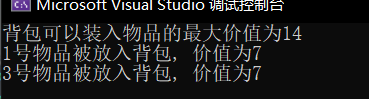空间优化
这里使用的二维数组实质上可以用一个一维数组代替，根据递推公式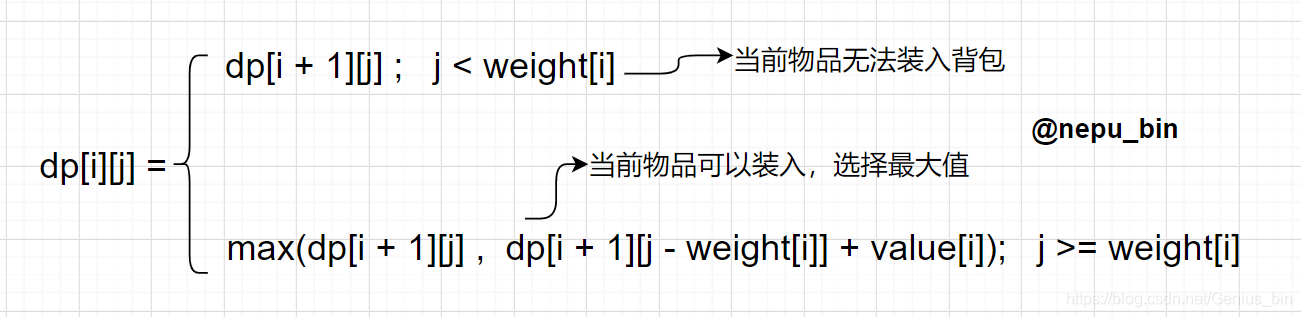当前背包的最大值取决于上一次遍历得到的最优解， 使用一维动态数组时需要注意：不能再从左至右遍历：
假设当前遍历的物品编号为i，weight[i] = 1，价值value[i] = 15, 且dp = 0;
如从左至右遍历：（dp[i] 表示 背包容量为 i 时取得的最大值）

dp = max(dp[1 - 1], dp[1 - weight[i] ] + value[i]) = dp + 15 = 15; 1 >= weight[i];
dp = max(dp[2 - 1], dp[2 - weight[i] ] + value[i]) = dp + 15 = 30;  2>=weight[i]
很显然这里对当前物品进行了重复装包，而在当前问题中这是不允许的，所以我们必须从右往左逆序遍历，可以根据递推公式尝试推导从右往左遍历时dp数组的取值情况（避免了重复取同一个物品）；

代码如下：
int ZeroOneKnapsack(vector<int>& weight, vector<int>& value, int c) {
int nums = weight.size(), col = c + 1;
vector<int> dp(c + 1, 0);//初始化一维数组dp长度为 c + 1且所有元素为0
int curNo = nums - 1;//当前遍历的物品编号
for (int i = nums - 1; i >= 0; i--) {//当前物品
for (int j = c; j >= weight[i]; j--) {//背包当前容量
dp[j] = max(dp[j], dp[j - weight[i]] + value[i]);
}
}
cout << dp[c] << endl;
return dp[c];
}

说明（小声bb）
科班大二在读，菜鸟一名，正修算法，博客主要用于记录自己对于一些经典题的理解和以及提升代码实现能力，均为课后根据思路（思路来源：上课老师讲解、课下查阅相关资料）原创完成，有表述不清或思路混乱的地方还请宽恕以及评论告知，一起学习一起进步，欢迎大家指正~~~


展开全文动态规划 算法 c++...